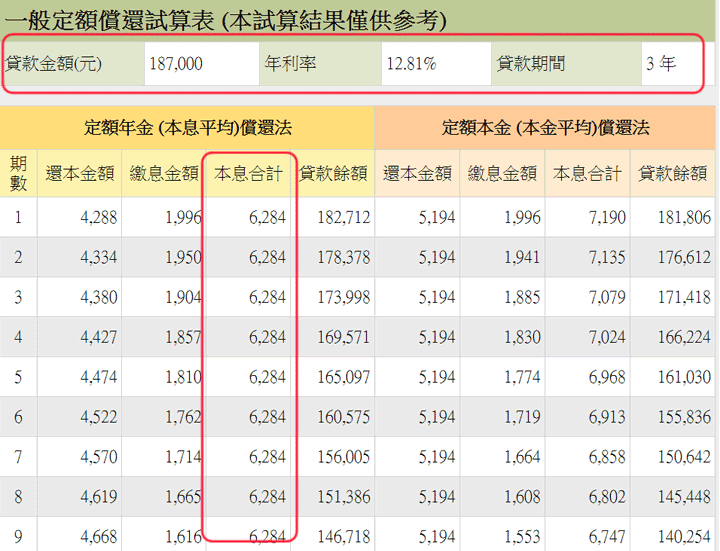﻿ 分期付款年利率試算

# 利用Excel的RATE函數計算

=RATE(nper, pmt, pv)

• nper：分期付款的期數
• pmt：每期本息金額 (因為是現金流出，必須用負值)
• pv：貸款金額

nper = 36, pmt = 6084, pv = 200000

=rate(36, -6084, 200000)
= 0.5% (月利率)

=0.5%*12 = 6%

# 考慮增列之費用

pv = 200000-(10000+3000) = 187,000
pmt =-(6084+200) = -6284
=RATE(36,-6284, 187000)*12 = 12.81%

# 等值年利率試算

## 線上試算

 貸款金額 元 (必填) 預扣費用之總額 元 (撥款時預先扣除之金額) 貸款月數 月(必填) 每月本息還款金額 元 (必填) 每月增加費用 元 實質年利率 總繳利息

# 驗證結果﻿
﻿

■ 本網站內容儘可能精確完整，但不保證無誤。若做為投資依據，風險請自行斟酌 ，本網站不負賠償之責任。
■ 網站所有資料均為版權所有，非經書面允許請勿轉載或使用。